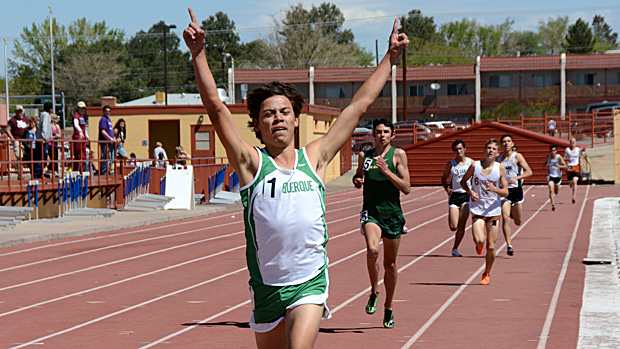# 5A Boys 2014?Andres Franco, shown here winning the 1600 at the Albuquerque Invitational, projects to be among the top athletes in 5A boys for 2014. Photo by Blake Wood.

So now that the 2013 outdoor track and field season has come and gone, what of 2014? You might be interested to know that, at least as of this moment, Cleveland does not appear to the quite as dominant of a force next year as this year. Graduation will do that to you know and then. That's not to say Cleveland is going away any time soon, only to suggest that there is some light at the end of the tunnel for schools wanting to hoist a track and field trophy of their own. Cibola would appear to be the first on the list of said schools.

If we take this year's best marks from our database, toss out the relays, and score those marks as a meet, here's what we get for 5A boys:

### 2013 NM HS M Outdoor Track Team Scores (Returners Only)

Calculated as of May 22, 2013

These rankings were calculated by scoring the rankings as a 10-8-6-5-4-3-2-1 meet without relays.

Athletes whose graduation years are unknown were left out of the list.

 1. Sue Cleveland High School 100 Aaron Flores (1600m 3 + 3200m 10 = 13) Brandon Leitch (J 4 = 4) Camden Davis (200m 1 + 400m 8 + LJ 8 = 17) Casey Shearer (PV 1.5 = 1.5) Jacob Anderson (D 8 = 8) Jacob Earnest (HJ 1 = 1) Jared Mayoral (1600m 4 + 3200m 8 + 800m 4 = 16) Keaton Frieberg (110H 2 + 300H 5 = 7) Kian Homme (J 3 = 3) Kyle Petrey (TJ 6 = 6) Michael Roseborough (D 6 = 6) Nathan Munoz (100m 1 + 110H 5 = 6) Tristan Bem (PV 1.5 = 1.5) Tyler Chavez (300H 6 + HJ 4 = 10) 2. Cibola High School 87 Alexander Armenta (HJ 4 = 4) Blair Manly (400m 3 = 3) Chris Harlow (1600m 1 + 3200m 6 = 7) Christopher Garcia (J 8 = 8) Frankie Scorfina (1600m 10 + 3200m 2 + 800m 10 = 22) Ivan Cornelius (100m 4.5 + 200m 10 + LJ 4.5 = 19) Juan Pimentel (D 5 + S 8 = 13) Marcell Powdrell (110H 1 = 1) Ryan Stebbins (100m 10 = 10) 3. Hobbs High School 59.5 Brandon Amaya (100m 8 + 200m 3 = 11) Cameron Kemp (J 5 = 5) Cris Sharp (3200m 4 = 4) Dakota Jones (PV 8 = 8) Devonte Brown (HJ 10 = 10) Kolin Zembas (110H 10 + 300H 0.5 = 10.5) Oscar Juardo (TJ 5 = 5) Samuel Carrasco (800m 6 = 6) 4. Clovis High School 56.5 Darrian Whiting (400m 5 = 5) Hector Flores (PV 5 = 5) Kamal Cass (LJ 10 + TJ 10 = 20) Kenny Robinson (D 4 + S 2 = 6) Pedro Acuna (S 5 = 5) Taylor Stratton (300H 3 = 3) Traivon Sopila (100m 4.5 + HJ 4 = 8.5) Tucker Dobbs (400m 4 = 4) 5. Rio Rancho High School 55 Anthony Palmeri (D 3 = 3) Cj Williams (100m 3 + 200m 4 + LJ 1 = 8) Colby Stinson (D 1 + S 10 = 11) Matt Guevara (J 2 = 2) Matt Jackson (110H 8 + LJ 6 = 14) Richie Getchell (PV 5 = 5) Tristen Foley (200m 2 + 400m 10 = 12) 6. Volcano Vista High School 37 Matthew Andrew (400m 1 = 1) Mike Budick (D 10 + J 6 + S 6 = 22) Noah Madrid (S 4 = 4) Robert Peele (200m 6 = 6) Taran Robinson (TJ 4 = 4) 6. Las Cruces High School 37 Andrew Thatcher (110H 4 + 300H 8 = 12) Isaiah McIntyre (110H 6 + 300H 10 + HJ 7 + LJ 2 = 25) 8. Manzano High School 23 Chris Ramirez (300H 2 = 2) Hayden Walker (PV 10 = 10) Justin Kerstetter (1600m 8 + 3200m 1 = 9) Sean Reaves (100m 2 = 2) 9. Eldorado High School 21 Adam Monroe (800m 5 = 5) Anthony Schluter (S 3 = 3) Taylor Potter (1600m 6 + 3200m 5 = 11) Tim Cutlip (800m 1 + HJ 1 = 2) 10. Alamogordo High School 20.5 Archie Hatol (PV 5 = 5) Kevin Patterson (PV 1.5 = 1.5) Mark Haywood (200m 5 + 400m 6 = 11) Seth Torres (TJ 3 = 3) 11. Mayfield High School 15 Isaac Figueroa (HJ 7 + TJ 8 = 15) 11. Sandia High School 15 Corey Smith (400m 2 = 2) Jeremiah Thompson (D 2 + S 1 = 3) Malik Gordon (J 10 = 10) 13. Atrisco Heritage Academy 14 Dante Hernandez (100m 6 = 6) Gilbert Ramirez (800m 8 = 8) 14. La Cueva High School 13 Brett Fenton (1600m 2 = 2) Gus Swanson (110H 3 + 300H 4 = 7) Stephen Criel (HJ 1 + LJ 3 = 4) 15. Carlsbad High School 12.5 Cody Tanner (200m 8 + LJ 4.5 = 12.5) 16. Albuquerque High School 11 Andres Franco (1600m 5 + 3200m 3 + 800m 3 = 11) 17. Highland High School 2 Jose Luis Ortega (TJ 2 = 2) 17. Rio Grande High School 2 Paul Chavez (J 1 = 1) Rafael De La Rosa (TJ 1 = 1) 17. Onate High School 2 Wiley Hughes (800m 2 = 2) 20. West Mesa High School 1.5 Matt Montoya (PV 1.5 = 1.5) 21. Gadsden High School 0.5 Gustavo Alvidrez (300H 0.5 = 0.5)